# Integral of arcsin(x)

In this post I am going to show you how to integrate the inverse function of sin(x), arcsin(x), step-by-step. But first, let’s clear a few things.

What is arcsin(x)?

Arcsine is the inverse function of sine, therefore: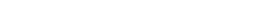These are equivalent forms: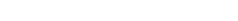The last form looks a lot like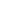, which is equal to 1/x. In the case of trigonometric functions though, it just means inverse function, not one over that function. So,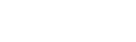but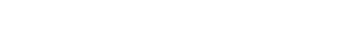Anyway, we are now ready to integrate!

Integral of arcsin(x)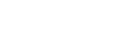The technique required for this integral is integration by parts:

Since we want to solve the integral of arcsin(x), it would make no sense to let dv=arcsin(x)dx. Also, arcsin(x) is the same as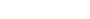, so we can integrate 1 and differentiate arcsin(x), whose derivative is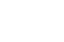.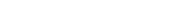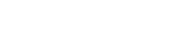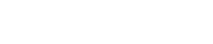Now we can use u-substitution, letting 1-x² be our u. When differentiating this, it will generate an x that cancels out with the one at the numerator; since we already used u in integration by parts, we will use t.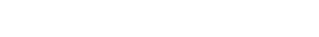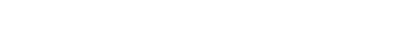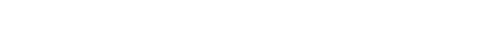Let’s now undo the substitution (remember that t=1-x²):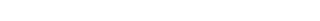Overall: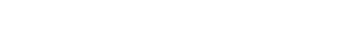And that’s it! Now try to solve this definite integral and leave the answer in the comments!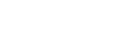I hope everything was clear and if you have any questions leave a comment and I’ll be happy to help!

Join 31 other subscribers.